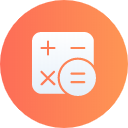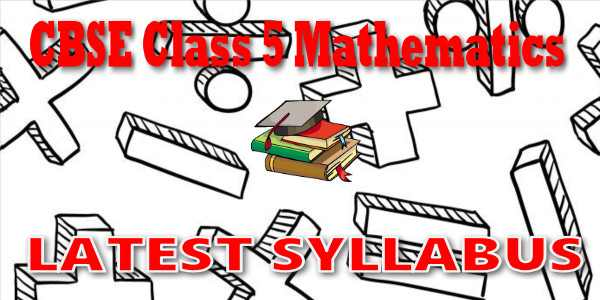No products in the cart.

# CBSE - Class 05 - Mathematics - CBSE Syllabus## CBSE, JEE, NEET, CUET

Question Bank, Mock Tests, Exam Papers

NCERT Solutions, Sample Papers, Notes, Videos

## CBSE Syllabus for Class 05 Mathematics

CBSE Syllabus Class 5 Mathematics### Student Subscription

##### Unlock the exclusive content designed for the toppers## myCBSEguide App

Complete Guide for CBSE Students

NCERT Solutions, NCERT Exemplars, Revison Notes, Free Videos, CBSE Papers, MCQ Tests & more.

# Latest CBSE Syllabus for Class 5 MathematicsCBSE syllabus for class 5 Mathematics 2018, 2019, 2020, 2021, 2022 as per cbse.nic.in new curriculum. CBSE syllabus is available for free download in PDF format. Download latest CBSE syllabus of 5th Mathematics as PDF format. Mathematics syllabus for cbse class 5 is also available in myCBSEguide app, the best app for CBSE students.

## CBSE Academics Unit - Curriculum Syllabus

CBSE has special academics unit to design curriculum and syllabus. The syllabus for CBSE class 5 Mathematics is published by cbse.nic.in Central Secondary Education, Head Office in New Delhi. The latest syllabus for class 5 Mathematics includes list of topics and chapters in Mathematics. CBSE question papers are designed as per the syllabus prescribed for current session.

## CBSE Syllabus category

Secondary School Curriculum (class 9 and class 10)

• Senior School Curriculum (class 11 and class 12)
• Vocational Courses (Class 11 and class 12)

CBSE Class V
Mathematics
CBSE Syllabus

Geometry (16 hrs.)
SHAPES & SPATIAL UNDERSTANDING

• Gets the feel of perspective while drawing a 3-D object in 2-D.
• Gets the feel of an angle through observation and paper folding.
• Identifies right angles in the environment.
• Classifies angles into right, acute and obtuse angles.
• Represents right angle, acute angle and obtuse angle by drawing and tracing.
• Explores intuitively rotations and reflections of familiar 2-D shapes.
• Explores intuitively symmetry in familiar 3-D shapes.
• Makes the shapes of cubes, cylinders and cones using nets especially designed for this purpose.

Numbers (40 hrs.)
NUMBERS AND OPERATIONS

• Finds place value in numbers beyond 1000.
• Appreciates the role of place value in addition, subtraction and multiplication algorithms.
• Uses informal and standard division algorithms.
• Explains the meaning of factors and multiples.

MENTAL ARITHMETIC

• Estimates sums, differences, products and quotients and verifies using approximation.

FRACTIONAL NUMBERS

• Finds the fractional part of a collection.
• Compares fractions.
• Identifies equivalent fractions.
• Estimates the degree of closeness of a fraction to known fractions (12,14,34etc)(12,14,34etc)
• Uses decimal fractions in the context of units of length and money.
• Expresses a given fraction in decimal notation and vice versa.

Money (5 hrs.)

• Applies the four operations in solving problems involving money.

Measurement (26 hrs.)
LENGTH

• Determines area and perimeter of simple geometrical figures.
• Applies the four operations in solving problems involving length, weight and volume.
• Relates commonly used larger and smaller units of length, weight and volume and converts one to the other.
• Applies simple fractions to quantities.
• Converts fractional larger unit into complete smaller units.
• Appreciates volume of a solid body: intuitively and also by informal measurement.
• Uses addition and subtraction in finding time intervals in simple cases.

Data Handling (6 hrs.)

• Collects two-dimensional quantitative data represents the data in the form of a table.
• Draws a bar graph or a pictograph to present a data.

Patterns (6 hrs.)

• Identifies patterns in square numbers, triangular numbers.
• Relates sequences of odd numbers between consecutive square numbers.
• Makes border strip and tiling patterns.

## CURRICULUM / SYLLABUS OF VOCATIONAL COURSES

CURRICULUM / SYLLABUS

 Scheme of Studies and List of Vocational Courses Application form to offer vocational courses Secondary School Curriculum (Under NSQF) Senior School Curriculum (Vocational)## myCBSEguide

Trusted by 1 Crore+ Students

#### Question Paper Creator

• Create papers in minutes
• Print with your name & Logo
• 5 Lakhs+ Questions
• Solutions Included
• Based on CBSE Syllabus
• Best fit for Schools & Tutors## Test Generator

Create papers at ₹10/- per paper## CUET Mock Tests

75,000+ questions to practice only on myCBSEguide app